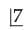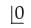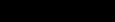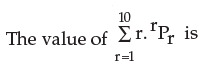Test: Basic Concepts Of Permutations And Combinations- 1

# Test: Basic Concepts Of Permutations And Combinations- 1

Test Description

## 40 Questions MCQ Test Business Mathematics and Logical Reasoning & Statistics | Test: Basic Concepts Of Permutations And Combinations- 1

Test: Basic Concepts Of Permutations And Combinations- 1 for CA Foundation 2023 is part of Business Mathematics and Logical Reasoning & Statistics preparation. The Test: Basic Concepts Of Permutations And Combinations- 1 questions and answers have been prepared according to the CA Foundation exam syllabus.The Test: Basic Concepts Of Permutations And Combinations- 1 MCQs are made for CA Foundation 2023 Exam. Find important definitions, questions, notes, meanings, examples, exercises, MCQs and online tests for Test: Basic Concepts Of Permutations And Combinations- 1 below.
Solutions of Test: Basic Concepts Of Permutations And Combinations- 1 questions in English are available as part of our Business Mathematics and Logical Reasoning & Statistics for CA Foundation & Test: Basic Concepts Of Permutations And Combinations- 1 solutions in Hindi for Business Mathematics and Logical Reasoning & Statistics course. Download more important topics, notes, lectures and mock test series for CA Foundation Exam by signing up for free. Attempt Test: Basic Concepts Of Permutations And Combinations- 1 | 40 questions in 40 minutes | Mock test for CA Foundation preparation | Free important questions MCQ to study Business Mathematics and Logical Reasoning & Statistics for CA Foundation Exam | Download free PDF with solutions
 1 Crore+ students have signed up on EduRev. Have you?
Test: Basic Concepts Of Permutations And Combinations- 1 - Question 1

### Choose the most appropriate option (a) (b) (c) or (d) 4P3 is evaluated as

Detailed Solution for Test: Basic Concepts Of Permutations And Combinations- 1 - Question 1

4P3

= 4!/(4!-3!)

= (4!)/1!

= 4*3*2*!

= 24

Test: Basic Concepts Of Permutations And Combinations- 1 - Question 2

### 4P4 is equal to

Test: Basic Concepts Of Permutations And Combinations- 1 - Question 3

###is equal to

Test: Basic Concepts Of Permutations And Combinations- 1 - Question 4is a symbol equal to

Detailed Solution for Test: Basic Concepts Of Permutations And Combinations- 1 - Question 4

0! = 1

Test: Basic Concepts Of Permutations And Combinations- 1 - Question 5

In nPr, n is always

Test: Basic Concepts Of Permutations And Combinations- 1 - Question 6

In nPr , the restriction is

Test: Basic Concepts Of Permutations And Combinations- 1 - Question 7

In nPr = n (n–1) (n–2) ………………(n–r–1), the number of factor is

Test: Basic Concepts Of Permutations And Combinations- 1 - Question 8

nPr can also written as

Test: Basic Concepts Of Permutations And Combinations- 1 - Question 9

If nP4 = 12 × nP2, the n is equal to

Test: Basic Concepts Of Permutations And Combinations- 1 - Question 10

If . nP3 : nP2 = 3 : 1, then n is equal to

Test: Basic Concepts Of Permutations And Combinations- 1 - Question 11

m+nP2 = 56, m–nP2 = 30 then

Test: Basic Concepts Of Permutations And Combinations- 1 - Question 12

If  5Pr = 60, then the value of r is

Test: Basic Concepts Of Permutations And Combinations- 1 - Question 13

If n1+n2P2 = 132, n1–n2P2 = 30 then,

Test: Basic Concepts Of Permutations And Combinations- 1 - Question 14

The number of ways the letters of the word COMPUTER can be arranged is

Detailed Solution for Test: Basic Concepts Of Permutations And Combinations- 1 - Question 14

Since all letters in the word "COMPUTER" are distinct then the arrangements is 8! = 8 x 7 x 6 x 5 x 4 x 3 x 2 x 1 = 40320

Test: Basic Concepts Of Permutations And Combinations- 1 - Question 15

The number of arrangements of the letters in the word FAILURE, so that vowels are always coming together is

Test: Basic Concepts Of Permutations And Combinations- 1 - Question 16

10 examination papers are arranged in such a way that the best and worst papers never come together. The number of arrangements is

Detailed Solution for Test: Basic Concepts Of Permutations And Combinations- 1 - Question 16

No. of ways in which 10 paper can arrange is 10! Ways.
When the best and the worst papers come together, regarding the two as one paper, we have only 9 papers.
These 9 papers can be arranged in 9! Ways.
And two papers can be arranged themselves in 2! Ways.
No. of arrangement when best and worst paper do not come together,
= 10!- 9!.2! = 9!(10-2) = 8.9!.

Test: Basic Concepts Of Permutations And Combinations- 1 - Question 17

n articles are arranged in such a way that 2 particular articles never come together. The number of such arrangements is

Detailed Solution for Test: Basic Concepts Of Permutations And Combinations- 1 - Question 17

N. particles arrange in a way = n!

if two particles are arranged together then
there is only (n-1) particles

when two particles are together = (n-1)!

and two particles are arranged in= 2! ways

Thus, number of ways in which no particles
are not come together

=n! -2×(n-1)!

=( n-1)!×(n-2)

Test: Basic Concepts Of Permutations And Combinations- 1 - Question 18

If 12 school teams are participating in a quiz contest, then the number of ways the first, second and third positions may be won is

Test: Basic Concepts Of Permutations And Combinations- 1 - Question 19

The sum of all 4 digit number containing the digits 2, 4, 6, 8, without repetitions is

Test: Basic Concepts Of Permutations And Combinations- 1 - Question 20

The number of 4 digit numbers greater than 5000 can be formed out of the  digits 3,4,5,6 and 7(no. digit is repeated). The number of such is

Test: Basic Concepts Of Permutations And Combinations- 1 - Question 21

4 digit numbers to be formed out of the figures 0, 1, 2, 3, 4 (no digit is repeated) then number of such numbers is

Test: Basic Concepts Of Permutations And Combinations- 1 - Question 22

The number of ways the letters of the word “Triangle” to be arranged so that the word ’angle’ will be always present is

Test: Basic Concepts Of Permutations And Combinations- 1 - Question 23

If the letters word ‘Daughter ’ are to be arranged so that vowels occupy the odd places, then number of different words are

Detailed Solution for Test: Basic Concepts Of Permutations And Combinations- 1 - Question 23

There are only 3 vowels, a, u, and e, and there are 4 odd places.  So
one of the odd spaces must contain a consonant.
We can choose 3 of the 4 odd places to put the vowels in C(4,3) or 4 ways.
For each of those vowel , we can  rearrange them  in P(3,3) or 3! or 6 ways.
And we can rearrange the 5 consonants in P(5,5) or 5! or 120 ways.
Hence the total number of different words formed are 6*4*120 = 2880

Test: Basic Concepts Of Permutations And Combinations- 1 - Question 24

The number of ways in which 7 girls form a ring is

Test: Basic Concepts Of Permutations And Combinations- 1 - Question 25

The number of ways in which 7 boys sit in a round table so that two particular boys may sit together is

Detailed Solution for Test: Basic Concepts Of Permutations And Combinations- 1 - Question 25

No. of ways in which n people can sit in a round table = (n-1)!
So to make them sit together, consider them as a single unit in the sitting arrangement ie. n= 6.
Number of ways so that two particular boys may sit together in the round table:-

(6 -1)!*2

(The two particular boys can also be switched among themselves)

=> 120*2
=> 240

Test: Basic Concepts Of Permutations And Combinations- 1 - Question 26

In how many ways can 10 examination papers be arranged so that the best and the worst papers never come together?

Detailed Solution for Test: Basic Concepts Of Permutations And Combinations- 1 - Question 26

No. of ways in which 10 paper can arranged is 10! Ways.
When the best and the worst papers come together, regarding the two as one paper, we have only 9 papers.
These 9 papers can be arranged in 9! Ways.
And two papers can be arranged themselves in 2! Ways.
No. of arrangement when best and worst paper do not come together,
= 10! - 9! × 2!
= 9!(10 - 2)
= 8 × 9!

Test: Basic Concepts Of Permutations And Combinations- 1 - Question 27

3 ladies and 3 gents can be seated at a round table so that any two and only two of the ladies sit together. The number of ways is

Test: Basic Concepts Of Permutations And Combinations- 1 - Question 28

The number of ways in which the letters of the word DOGMATIC can be arranged is

Test: Basic Concepts Of Permutations And Combinations- 1 - Question 29

The number of arrangements of 10 different things taken 4 at a time in which one particular thing always occurs is

Detailed Solution for Test: Basic Concepts Of Permutations And Combinations- 1 - Question 29

Number of different things = 10

Numbers of things which are taken at a time = 4

Now, it is said that one particular thing always occur.

So The question reduces to selecting 9 things from the 3 things

This can be done easily by using the concept of combinations

Number of ways selecting 3 things out of 9 different things such that one particular thing always occur =Now, the 4th thing is fixed so number of ways for selecting the 4th thing = 4! = 24

So, Required number of ways = 84 × 24 = 2016

Test: Basic Concepts Of Permutations And Combinations- 1 - Question 30

The number of permutations of 10 different things taken 4 at a time in which one particular thing never occurs is

Test: Basic Concepts Of Permutations And Combinations- 1 - Question 31

Mr. X and Mr. Y enter into a railway compartment having six vacant seats. The number of ways in which they can occupy the seats is

Test: Basic Concepts Of Permutations And Combinations- 1 - Question 32

The number of numbers lying between 100 and 1000 can be formed with the digits 1, 2, 3, 4, 5, 6, 7 is

Test: Basic Concepts Of Permutations And Combinations- 1 - Question 33

The number of numbers lying between 10 and 1000 can be formed with the digits 2,3,4,0,8,9 is

Detailed Solution for Test: Basic Concepts Of Permutations And Combinations- 1 - Question 33

Two digits number (0 can't come on unit place) 5 × 5 = 25
Three digits number 5 × 5 × 4 = 100
Hence the total number of numbers between 10 and 1000 that can be formed using given digits is = 125

Test: Basic Concepts Of Permutations And Combinations- 1 - Question 34

In a group of boys the number of arrangement of 4 boys is 12 times the number of arrangements of 2 boys. The number of boys in the group is

Test: Basic Concepts Of Permutations And Combinations- 1 - Question 35Test: Basic Concepts Of Permutations And Combinations- 1 - Question 36

The total number of 9 digit numbers of different digits is

Test: Basic Concepts Of Permutations And Combinations- 1 - Question 37

The number of ways in which 6 men can be arranged in a row so that the particular 3 men sit together, is

Test: Basic Concepts Of Permutations And Combinations- 1 - Question 38

There are 5 speakers A, B, C, D and E. The number of ways in which A will speak always before B is

Test: Basic Concepts Of Permutations And Combinations- 1 - Question 39

There are 10 trains plying between Calcutta and Delhi. The number of ways in which a person can go from Calcutta to Delhi and return by a different train is

Test: Basic Concepts Of Permutations And Combinations- 1 - Question 40

The number of ways in which 8 sweets of different sizes can be distributed among 8 persons of different ages so that the largest sweat always goes to be younger assuming that each one of then gets a sweet is

Detailed Solution for Test: Basic Concepts Of Permutations And Combinations- 1 - Question 40

The number of ways in which 8 sweets of different sizes can be distributed among 8 persons of different ages so that the largest sweat goes to the younger assuming that each one of them gets a sweet.
Since the largest goes to the youngest, that leaves 7 sweets  to distribute to 7 people.

7P7 = 7! = 5040 ways

## Business Mathematics and Logical Reasoning & Statistics

131 videos|149 docs|87 tests
 Use Code STAYHOME200 and get INR 200 additional OFF Use Coupon Code
Information about Test: Basic Concepts Of Permutations And Combinations- 1 Page
In this test you can find the Exam questions for Test: Basic Concepts Of Permutations And Combinations- 1 solved & explained in the simplest way possible. Besides giving Questions and answers for Test: Basic Concepts Of Permutations And Combinations- 1, EduRev gives you an ample number of Online tests for practice

## Business Mathematics and Logical Reasoning & Statistics

131 videos|149 docs|87 tests